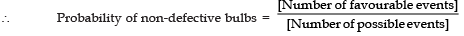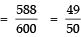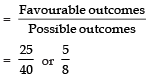Courses

# Value Based Questions- Probability Class 10 Notes | EduRev

## Class 10 : Value Based Questions- Probability Class 10 Notes | EduRev

The document Value Based Questions- Probability Class 10 Notes | EduRev is a part of the Class 10 Course Class 10 Mathematics by VP Classes.
All you need of Class 10 at this link: Class 10

VALUE BASED QUESTIONS

Q1. Roshan is a wholesaler of electric-bulbs. He sells a box of 600 electric bulbs which contains 12 defective bulbs to an electrician. He gives 12 extra bulbs to the electrician. In the process of replacing the defective bulbs by non-defective bulbs, the electrician takes out one bulb at random from the box.
(a) What is the probability that it is non-defective bulb?
(b) Which mathematical concept is used in the above problem?
(c) By giving 12 extra non-defective bulbs to the electrician, which value is depicted by Roshan?

Sol. (a) Total number of bulbs in the box = 600
Number of defective bulbs = 12
Number of non-defective bulbs = 600 – 12 = 588
Number of possible events = 600
Number of favourable events = 588(b) Probability
(c) Honesty

Q2. There are 40 students in class X, of whom 25 are girls and 15 are boys. They plan to go to help the earthquake victims in a camp. Their class-teacher has to select one student at random for the class representative.
(a) What is the probability that the student selected for class-representative is (i) a girl (ii) a boy
(b) Which mathematical concept is used in the above problem?
(c) By planning to go for the help of earthquake victims, which value is depicted by the students of class X?

Sol. (a) Total number of students in the class = 40
Number of boys = 15 Number of girls = 25
(i) Since, the class representative selected at random is to be a girl
∴ Number of possible outcomes = 40
Number of favourable outcomes = 25
[∵ There are 25 girls in the class]
∴ Probability of a girl to be the representative(ii) Since, the class-representative selected at random is to be a boy
∴ Number of favourable outcomes = 15

⇒ Probability of a boy to be the representative =(b) Probability
(c) Helping the persons, in need.

Offer running on EduRev: Apply code STAYHOME200 to get INR 200 off on our premium plan EduRev Infinity!

,

,

,

,

,

,

,

,

,

,

,

,

,

,

,

,

,

,

,

,

,

;